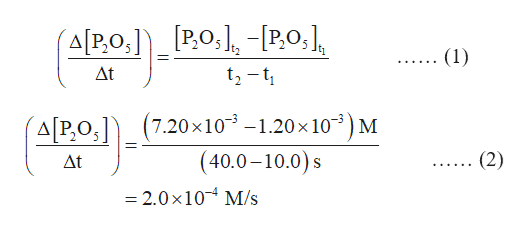# Consider the reaction2H3PO4→P2O5+3H2OUsing the information in the following table, calculate the average rate of formation of P2O5 between 10.0 and 40.0 s.Time (s)010.020.030.040.050.0[P2O5] (M)01.20×10−34.20×10−36.00×10−37.20×10−37.80×10−3Express your answer with the appropriate units.

Question
1119 views

Consider the reaction

2H3PO4→P2O5+3H2O

Using the information in the following table, calculate the average rate of formation of P2O5 between 10.0 and 40.0 s.

 Time (s) 0 10.0 20.0 30.0 40.0 50.0 [P2O5] (M) 0 1.20×10−3 4.20×10−3 6.00×10−3 7.20×10−3 7.80×10−3
check_circle

Step 1

For the given reaction,

2H3PO4   ------à P2O5 + 3H2O

The expression for rate of disappearance of reactants and appearance of products is:

Step 2

The average rate of formation of P2O5 between 10.0 s and 40.0 s is calculated by the expression given in...help_outlineImage Transcriptionclose(АРo) _[РО,1, - РО,], tz-t (1) t+** в At АРО) (720х10"-1.20х10") м (40.0-10.0)s X (2) At — 2.0х10 М/s fullscreen

### Want to see the full answer?

See Solution

#### Want to see this answer and more?

Solutions are written by subject experts who are available 24/7. Questions are typically answered within 1 hour.*

See Solution
*Response times may vary by subject and question.
Tagged in